# GRE Quantitative Reasoning - Exponents: Tutoring Solution Chapter Exam

Exam Instructions:

Choose your answers to the questions and click 'Next' to see the next set of questions. You can skip questions if you would like and come back to them later with the yellow "Go To First Skipped Question" button. When you have completed the practice exam, a green submit button will appear. Click it to see your results. Good luck!

### Page 1

#### Question 1 1. Simplify the expression using positive exponents.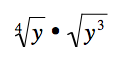#### Question 2 2. Which of the following is equivalent to the following expression?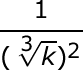#### Question 3 3. Simplify the following expression.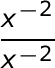### Page 2

#### Question 6 6. Solve the exponential equation below for x: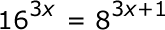#### Question 8 8. Simplify the expression below using only positive exponents.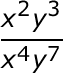#### Question 9 9. Simplify the following expression using positive exponents.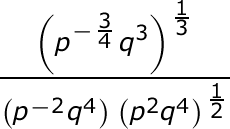#### Question 10 10. Which of the following is equivalent to the following expression?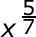### Page 3

#### Question 11 11. Which of the following is equivalent to the expression below?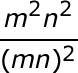#### Question 12 12. Simplify this expression using positive exponents.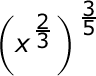#### Question 14 14. Simplify the following expression using positive exponents only.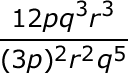#### Question 15 15. Simplify the following expression.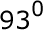### Page 4

#### Question 16 16. Simplify the expression below using positive exponents only.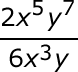#### Question 17 17. Solve, to 2 decimal places, the exponential equation below for x: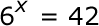#### Question 18 18. Simplify the expression below.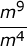#### Question 19 19. Using positive exponents, simplify the expression.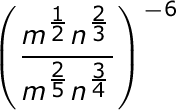### Page 5

#### Question 21 21. Simplify using ONLY positive exponents.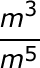#### Question 22 22. Simplify using ONLY positive exponents.#### Question 24 24. Simplify the expression below using positive exponents.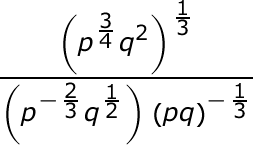#### Question 25 25. Which of the following is equivalent to the expression below?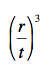### Page 6

#### Question 26 26. Solve the exponential equation below for x: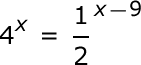#### Question 27 27. Which of the following is equivalent to the expression below?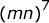#### Question 28 28. Which of the following exponential expressions is equal to this radical expression?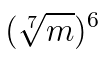#### Question 29 29. Which of the following would be true regarding the following exponential expression?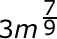#### Question 30 30. Simplify the following expression using positive exponents.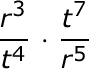#### GRE Quantitative Reasoning - Exponents: Tutoring Solution Chapter Exam Instructions

Choose your answers to the questions and click 'Next' to see the next set of questions. You can skip questions if you would like and come back to them later with the yellow "Go To First Skipped Question" button. When you have completed the practice exam, a green submit button will appear. Click it to see your results. Good luck!

Support